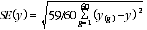4150.0 - Time Use Survey: User Guide, 2006
Latest ISSUE Released at 11:30 AM (CANBERRA TIME) 21/02/2008
Page tools:Print AllRSSSearch this Product
Contents >> Using the CURF >> Reliability of Estimates

RELIABILITY OF ESTIMATES

Two types of error, sampling error and non-sampling error, are possible in an estimate based on a sample survey. More information is available in Chapter 4 'Data Quality' in this User Guide.

Standard Errors (SEs) are one of the methods used to measure sampling variability. As mentioned in the section 'Use of weights' earlier in this chapter, each record on the CURFs contain 60 'replicate weights'. The SE for each estimate produced from the CURFs can be calculated using the replicate weights provided.

SEs can be calculated using what is known as the 60 group jackknife error estimator. When calculating SEs it is important to select the replicate weights which are most appropriate for the analysis being undertaken (see Appendix 2 for replicate weights at each level).

To obtain the SE of a weighted estimate y, calculate the same estimate using each of the 60 replicate weights. The variability between these replicate estimates (denoting y(g) for group number g) is used to measure the SE of the original weighted estimate y, using the formula:where

g = the replicate group number

y(g) = the weighted estimate, having applied the weights for replicate group g

y = the weighted estimate from the full sample

Use of the 60 group jackknife method for complex estimates, such as regression parameters from a statistical model, is not straightforward and may not be appropriate. The method as described does not apply to investigations where survey weights are not used, such as unweighted statistical modelling. For more information on the 60 group jackknife method of SE estimated, see Research Paper: Weighting and Standard Error Estimation for ABS Household Surveys (Methodology Advisory Committee), 1999 (Cat. no. 1352.0.55.029).

Information on calculating the relative standard error (RSE) of an estimate is available in Chapter 4 'Data Quality' in this User Guide.

CURF users should be aware that estimates produced from the CURFs will differ from those in the published data due to actions taken to preserve confidentiality on the TUS CURFs.

 Previous Page Next Page